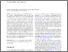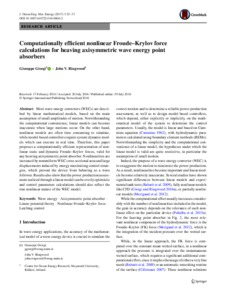# Computationally efficient nonlinear Froude–Krylov force calculations for heaving axisymmetric wave energy point absorbers

Giorgi, Giuseppe and Ringwood, John (2017) Computationally efficient nonlinear Froude–Krylov force calculations for heaving axisymmetric wave energy point absorbers. Journal of Ocean Engineering and Marine Energy, 3 (1). pp. 21-33. ISSN 2198-6444Previewmore...Add this article to your Mendeley library

## Abstract

Most wave energy converters (WECs) are described by linear mathematical models, based on the main assumption of small amplitudes of motion. Notwithstanding the computational convenience, linear models can become inaccurate when large motions occur. On the other hand, nonlinear models are often time consuming to simulate, while model-based controllers require system dynamic models which can execute in real time. Therefore, this paper proposes a computationally efficient representation of nonlinear static and dynamic Froude–Krylov forces, valid for any heaving axisymmetric point absorber. Nonlinearities are increased by nonuniform WEC cross sectional area and large displacements induced by energy maximising control strategies, which prevent the device from behaving as a wave follower. Results also show that the power production assessment realized through a linear model can be overly optimistic and control parameters calculations should also reflect the true nonlinear nature of the WEC model.

Item Type: Article Wave energy; Axisymmetric point absorber; Linear potential theory; Nonlinear Froude–Krylov force; Latching control; Faculty of Science and Engineering > Electronic EngineeringFaculty of Science and Engineering > Research Institutes > Centre for Ocean Energy Research 11776 https://doi.org/10.1007/s40722-016-0066-2 Professor John Ringwood 20 Nov 2019 17:35 Journal of Ocean Engineering and Marine Energy Springer YesItem control page